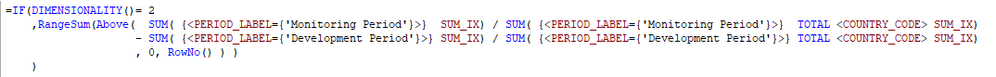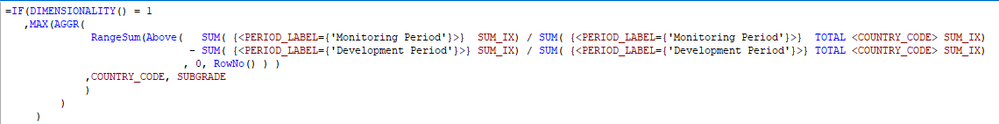# QlikView App Dev

Discussion Board for collaboration related to QlikView App Development.

cancel
Showing results for
Did you mean:Contributor II

## Help with RangeSum Above aggregation function

Hello,

I'm in desperate need of help since I've been trying several things but I can't make this work as it should so I would really appreciate it if someone could give me a hand.

I'm trying to calculate a statistic that gives the maximum difference between two columns that have the accumulated distribution (percentage) of a dimension. I could calculate the accumulated percentages and the difference using RangeSum Above but somehow when I try applying the max it doesn't work.

I attach the qvw and here is the pivot table I have:I am calculating the Accumulated diff like this:and the max like this:But as you see in the image it doesn't work in all cases.

Please find attached the qvw

Thanks!!!!

1 Solution

Accepted SolutionsMVP

Are you using QlikView 12 or above... if you are, then try this for Max

``````=IF(DIMENSIONALITY() = 1
,MAX(AGGR(
RangeSum(Above(   SUM( {<PERIOD_LABEL={'Monitoring Period'}>}  SUM_IX) / SUM( {<PERIOD_LABEL={'Monitoring Period'}>}  TOTAL <COUNTRY_CODE> SUM_IX)
- SUM( {<PERIOD_LABEL={'Development Period'}>} SUM_IX) / SUM( {<PERIOD_LABEL={'Development Period'}>} TOTAL <COUNTRY_CODE> SUM_IX)
, 0, RowNo() ) )
)
)
)

//     FirstSortedValue``````5 RepliesMVP

Are you using QlikView 12 or above... if you are, then try this for Max

``````=IF(DIMENSIONALITY() = 1
,MAX(AGGR(
RangeSum(Above(   SUM( {<PERIOD_LABEL={'Monitoring Period'}>}  SUM_IX) / SUM( {<PERIOD_LABEL={'Monitoring Period'}>}  TOTAL <COUNTRY_CODE> SUM_IX)
- SUM( {<PERIOD_LABEL={'Development Period'}>} SUM_IX) / SUM( {<PERIOD_LABEL={'Development Period'}>} TOTAL <COUNTRY_CODE> SUM_IX)
, 0, RowNo() ) )
)
)
)

//     FirstSortedValue``````Contributor II
Author

Thank you very much Sunny! It works now  😄

Yes, I'm using qlikview 12.20  I'm just wondering what does the  (SUBGRADE, (TEXT)  is doing?

Have a nice day 🙂MVP

Aggr() sort your dimensions in the load order which could be different from the order you are using in the chart. Why it makes a difference? It is because you are doing accumulation which is dependent on the order of dimension. In order to get the correct order... which it seems was the text sorting of your second dimension... we use this syntax. Syntax itself can be understood by reading the below article.Contributor II
Author

Great! I'm so thankful since I was quite frustrated after trying many different things and without your help, I don't think I would have been able to make it work. Thanks for your supportMVP

No problem at all